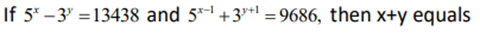### CAT 2019 – Slot 1 – Quantitative Ability –Let A and B be two regular polygons having a and b sides, respectively

Q. 1: Let A and B be two regular polygons having a and b sides, respectively. If b = 2a and each interior angle of B is 3/2 times each interior angle of A, then each interior angle, in degrees, of a regular polygon with a + b sides is

Each interior angle of n sided polygon = (n-2)*180/n

(b-2)*180/b = 3/2 (a-2)*180/a and b = 2a

So, 2a-2/2 = (a-2)*3/2

2a – 2 = 3a – 6

a = 4 and b = 8

So, a + b = 12, a 12 sided polygon

Each interior angle = (12-2)*180/12 = 150 degrees

Q. 2 :Given,

5^x – 3^y = 13438—–(1)

5^(x-1) + 3^(y+1) = 9686—-(2)

From equation 2, we get

5^x /5 + 3. 3^y = 9686

Multiplying equation 1 by 3 and adding, we get

5^x = 15625

So, x = 6

Putting x in equation 1, we get

3^y = 2187

Y = 7

So, x+y = 13

Q. 3: The number of common terms in the two sequences: 15, 19, 23, 27, 415 and 14, 19, 24, 29, , 464 is
1. 18
2. 19
3. 21
4. 20

Common difference of first series 15, 19, 23, 27…= 4

Common difference of 2nd series 14, 19, 24, 29 .. =5

So common difference of the series formed by common terms = 4*5 = 20

First common term = 19

So series = 19, 39 , 59, … last term < 415

nth term = 19+20(n-1) = 20n – 1 < 415

so 20n < 416

n < 416/20 = 20.8

so n = 20

Q. 4: Amal invests Rs 12000 at 8% interest, compounded annually, and Rs 10000 at 6% interest, compounded semi-annually, both investments being for one year. Bimal invests his money at 7.5% simple interest for one year. If Amal and Bimal get the same amount of interest, then the amount, in Rupees, invested by Bimal is

Total amount invested by Amal = 12000(1+0.08) + 10000(1+0.06/2)^2 = 12960+ 10609 = 23569

Bimal invests p amount at si

So, interest for bimal = p*7.5*1/100

Interest are same for a and b, so we get

23569-22000 = p*7.5*1/100

So, p = 20920

Inspiring Education… Assuring Success!!

Ghatkopar | Borivali | Andheri | Online